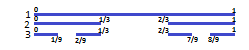# The Idea of the Georg Cantor Set

Cantor set, seen on the number line as the interval between 0 and 1 is an example of a fractal on the real number system as shown on the real number line. The Georg Cantor set is very easy and simple to construct just with the aid of a line that represent numbers where if one remove a section; it amounts to dealing with that part of the set.

Construction of Cantor set involves three steps which are outlined below:

1. Draw a horizontal number line that signifies the interval of real number system with the left and right endpoints labelled 0 and 1 respectively.

2.Cut off or wipe out or simply erase a section of this line that represents middle-thirds, that is between 1/3  and  2/3  of the drawn line segment. Once you have erased this middle-thirds section, you will be left with two thirds of the originally drawn number line.

3. Repeat step 2 on the two thirds of the original line that remains by erasing the middle-thirds from the two new lines that remains from step 2. If you repeat this step again a few times more, you will surely see a pattern that demonstrates a fractal.By applying iteration that is infinite on this set, we obtain a set of points that remain in the Cantor Set that are not thrown out in one of the middle-thirds intervals. If you plot a graph of this set pattern, the set will consist of particles of scattered points without any connection to the points of which the Cantor Set is made up.

Cantor expressed an important conclusion with this set pattern and that conclusion has to do with the fact that if certain functions are iterated continuously, they will produce effects that are similar to the Cantor Set. A good example is that of a function f(x)  = 4.5x (1-x) mapped over the real number line with its interval existing between 0 and 1. When the function f(x)  = 4.5x (1-x) is iterated, it will be observed that the some x-values that represent Cantor’s middle third are ‘’thrown’’ out of the interval between 0and 1.

The effect of this is that whatever is left will only be points within the Cantor Set when the function is iterated n times. This phenomenon will prove helpful in understanding Mandelbrot Set and other complex sets where issues of some points escaping the interval while some points remain within the interval will be better understood and appreciated.

0 Shares
Filed under Math.